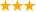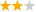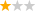# 奥克兰理工大学工程荣誉学士专业

## 01奥克兰理工大学工程荣誉学士专业解析

• 所属国家： 新西兰
• 大学： 奥克兰理工大学
• 课程学制： 4年
• 开学时间： 2月，7月
• 用户口碑： (0)条口碑
• 就业率： N/A
• 专业排名： N/A
• 学费： 39600.00纽币/年

## 02奥克兰理工大学工程荣誉学士专业介绍

1.Operations Research (OR) 运筹学
Operations Research一直以来被称之为“更好的科学”，它是一种制定决策的科学方法，主要研究如何让事情的周期变得更短，速度变得更快，成本变得更低，性能变得更可靠，利润变得更丰厚。运筹学的专业家建立了各种解决常见的复杂问题计算机模型，然后运用更高级的优化算法来指导他们制定最佳的决策。运筹学专业下的学生将更善于数学，计算以及统计这三个方面的技能，他们享受着成功解决数学谜题带来的快乐并为实际疑难问题提供最优化的解决方案。
2.Continuum Mechanics
Continuum Mechanics称之为连续介质力学，主要研究当外力作用于各种材料时所产生的现象。该专业主要包含两个方面的内容，固体力学和流体力学。

## 03奥克兰理工大学工程荣誉学士专业世界排名

 2022 2021 2020 2019 2018 2016 2017 2015 2014 2013 450+ 430+ 440+ 460+ 440+ 440+ N/A N/A N/A N/A

## 04奥克兰理工大学工程荣誉学士专业课程

Bachelor of Engineering (Honours)下共细分7个方向，每个方向的具体课程设置如下：
1.
Architectural Engineering Major - Bachelor of Engineering (Honours)

Year 1

Complete the following papers

Electrical Principles A (15 points)              Electrical Principles B (15 points)

Introduction to Engineering Design (15 points)  Engineering Mathematics I (15 points)

Engineering Materials I (15 points)            Mechanical Principles A (15 points)

Mechanical Principles B (15 points)           Introduction to Computing (15 points)

Year 2

Complete the following papers

Construction Materials (15 points)        Introduction to Structural Engineering (15 points) Quantity Surveying (15 points)           Building Construction I (15 points)

Construction Engineering Management I (15 points)   Engineering Mathematics II (15 points)

Engineering Design Methodology (15 points)         Solid Mechanics I (15 points)

Year 3

Complete the following papers

llumination Engineering (15 points)     Architectural Design and Sustainability (15 points)

Geotechnical Engineering (15 points)    Structural Analysis (15 points)

Architecture and Design Development I (15 points)  Heating, Ventilation and Air-conditioning Systems (HVAC) 15 points

Construction Engineering Management II (15 points)   Engineering Mathematics III (15 points)

Year 4

Complete the following papers

Structural Engineering Design (15 points)     Structural Dynamics (15 points)

Architectural Systems (15 points)         Architecture and Design Development II (15 points) Building Construction II (15 points)       Industrial Project (30 points)

Choose one of the following

Foundation Engineering (15 points)                Off-Site Construction (15 points)

Construction Equipment and Utilisation (15 points)    Construction Technology II (15 points) Architectural Acoustics (15 points)                 Fire Engineering Design (15 points)

2. Construction Engineering Major - Bachelor of Engineering (Honours)

Year 1

Students in all majors do the same papers in the first year.

Complete the following papers

Electrical Principles A (15 points)      Electrical Principles B (15 points)

Introduction to Engineering Design (15 points)  Engineering Mathematics I (15 points)

Engineering Materials I (15 points)     Mechanical Principles A (15 points)

Mechanical Principles B (15 points)     Introduction to Computing (15 points)

Year 2

Complete the following papers

Construction Materials (15 points)      Introduction to Structural Engineering (15 points)

Quantity Surveying (15 points)         Building Construction I (15 points)

Construction Engineering Management I (15 points)  Engineering Mathematics II (15 points)

Engineering Design Methodology (15 points)   Solid Mechanics I (15 points)

Year 3

Complete the following papers

Architectural Design and Sustainability (15 points)   Geotechnical Engineering (15 points)

Structural Analysis (15 points)           Concrete Structures (15 points)

Steel Structures (15 points)             Construction Planning (15 points)

Construction Engineering Management II (15 points)  Engineering Mathematics III (15 points)

Year 4

Complete the following papers

Structural Engineering Design (15 points)   Industrial Project (30 points)

And choose 60 points from the following

Structural Dynamics (15 points)           Architectural Systems (15 points)

Foundation Engineering (15 points)        Off-Site Construction (15 points)

Construction Equipment and Utilisation (15 points)  Construction Technology II (15 points)

3. Electrical and Electronic Engineering Major - Bachelor of Engineering (Honours)

Year 1

Complete the following papers

Electrical Principles A (15 points)      Electrical Principles B (15 points)

Introduction to Engineering Design (15 points)  Engineering Mathematics I (15 points)

Engineering Materials I (15 points)     Mechanical Principles A (15 points)

Mechanical Principles B (15 points)     Introduction to Computing (15 points

Year 2

Complete the following papers

Electronics (15 points)                 Signals and Systems (15 points)

Electronics Project (15 points)           Introduction to Microcontrollers (15 points)

Engineering Management I (15 points)    Engineering Mathematics II (15 points)

Engineering Mathematics III (15 points)   Circuit Theory (15 points)

Year 3

Complete the following papers

Communication Engineering (15 points)   Power Electronic Systems (15 points)

Instrumentation and Control Systems (15 points)   Power Systems Engineering (15 points)

Fields and Waves (15 points)            Design Project (15 points)

Embedded Digital Systems (15 points)  Engineering Numerical Techniques and Statistical Analysis (15 points)

Year 4

Complete the following papers

Engineering Management III (15 points)   Industrial Project (Electrical) (30 points)

Choose four papers from the following

Wireless Systems (15 points)            Electrical Machines and Drives (15 points)

Network Engineering (15 points)         Electrical Design and Protection (15 points)

Sustainable Energy Systems (15 points)    Computer Vision (15 points)

Advanced Control Systems (15 points)     System Identification and Adaptive Control (15 points)

Optimal Control (15 points)      Electrical Power Systems: Integrated Analysis (15 points)

Power Systems in Quality Management (15 points)   Specialist Readings B (15 points)

Specialist Readings D (15 points)          Digital Signal Processing (15 points)

Digital Systems Design (15 points)         Real Time Systems (15 points)

Embedded Software Engineering (15 points)

4. Maritime Engineering Majors - Bachelor of Engineering (Honours)

Year 1

Complete the following papers

Electrical Principles A (15 points)        Electrical Principles B (15 points)

Introduction to Engineering Design (15 points)   Engineering Mathematics I (15 points)

Engineering Materials I (15 points)       Mechanical Principles A (15 points)

Mechanical Principles B (15 points)      Introduction to Computing (15 points)

Year 2

Complete the following papers

Engineering Management I (15 points)    Engineering Mathematics II (15 points)

Hydrostatics (15 points)                Ship Design and Production (15 points)

Fluid Mechanics and Thermodynamics (15 points) Offshore and Maritime Engineering (15 points)

Solid Mechanics I (15 points)            Engineering Mathematics III (15 points)

Year 3 and 4

You transfer to the Australian Maritime College, University of Tasmania for Year 3 and 4 wher you study one of:

Marine and Offshore Engineering

Naval Architecture    Ocean Engineering    Marine and Offshore Engineering

Year 3:Covers a wide range of marine subjects such as mechanics, thermal energy, electrical powering systems.

Year 4:Apart from the year-long design and research project, you learn integrated process, applied control and maritime engineering design.

Naval Architecture

Year 3:Your study focuses on structural analysis, ship resistance, ship design and underwater vehicle technology.

Year 4:Apart from papers on advanced ship structures and computational fluid dynamics, your focus will be on a year-long design and research project.

Ocean Engineering

Year 3:You study aquaculture, concrete structures, deep water and finite element analysis.

Year 4:Covers coastal and subsea engineering as well as design of offshore structures. You also undertake a design and research project across the whole year.

Year 1

Students in all majors do the same papers in the first year. These papers cover mathematics and computational techniques, as well as the communication and teamwork skills that are essential for the team environment engineers work in.

5. Mechanical Engineering Major - Bachelor of Engineering (Honours)

Complete the following papers

Electrical Principles A (15 points)                   Electrical Principles B (15 points)

Introduction to Engineering Design (15 points)        Engineering Mathematics I (15 points)

Engineering Materials I (15 points)                  Mechanical Principles A (15 points)

Mechanical Principles B (15 points)## 09.奥克兰理工大学工程荣誉学士专业学生评价

课程难度 部分就读该课程的学生中途转专业甚至出现辍学，属较高难度课程类型。申请人应参考自身的学习能力谨慎选择。

## 提交评估，获取录取几率和2022年招生手册

•好评中评差评